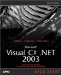# Creating Objects

To create an object, also called an instance , of a class, you use the new keyword. We've seen how this process works; you can see how we create a new object of the Calculator class in ch03_01.cs, Listing 3.1, and use that object's Addem method to add 2 and 3. Note the parentheses after Calculator in the statement Calculator obj = new Calculator(); . These parentheses are necessary when you use the new keyword. They let you pass data to a class's constructor , the special method that lets you initialize the data in a class. We'll go into depth about constructors later in this chapter.

##### Listing 3.1 Creating a New Class (ch03_01.cs)
` class ch03_01 {   static void Main()   {  Calculator obj = new Calculator();   System.Console.WriteLine("2 + 3 = {0}", obj.Addem(2, 3));  } } class Calculator {   public long Addem(int value1, int value2)   {     return value1 + value2;   } } `

Here's what you see when you run this example, ch03_01:

` `
` C:\>ch03_01 2 + 3 = 5 `

In C#, all objects are based on the System.Object class, which means that they already support the methods built into that class. You can see those methods in Table 3.1. Note in particular the ToString method, which returns a string representation of an object. You can customize this method to return a string for your own classes by overriding this method, as we'll see in the next chapter.

## FOR C++ PROGRAMMERS

In C#, all objects are based on the .NET System.Object class, and so support the properties and methods of that class.

##### Table 3.1. Public Methods of System.Object Objects

METHOD

MEANS

Equals

Indicates whether two Object instances are equal.

GetHashCode

Serves as a hash function for a particular type.

GetType

Returns the Type of the current instance.

ReferenceEquals

Determines whether the specified Object instances are the same instance.

ToString

Returns a String that represents the current Object .

It's worth mentioning that you can also create simple variables using new as well, because even those simple types are based on the System.Object class and have their own constructors. Here's an example:

` `
` int int1 = new int(); int int2 = new int(5);   //Initialize int2 to 5 `

The first statement here creates a new int variable named int1 . Remember that you can't use initialized variables in C#; in this case, int1 is automatically initialized to 0. In other words, the first statement above is logically identical to:

` `
` int int1 = 0; `

You can see the default values of the various value types created when you use the new operator in Table 3.2. Note that, although you can't set reference types to a default value of 0 as you see for value types in Table 3.2, their default value is null (which means they do not reference an object on the heap).

##### Table 3.2. Default Values of Value Types

VALUE TYPE

DEFAULT VALUE

bool

false

byte

char

'\0'

decimal

0.0M

double

0.0D

enum

The value of the expression ( E )0 (where E is the enum identifier)

float

0.0F

int

long

0L

sbyte

short

struct

The value produced by setting all value-type fields to their default values and all reference-type fields to null

uint

ulong

ushortMicrosoft Visual C#.NET 2003 Kick Start
ISBN: 0672325470
EAN: 2147483647
Year: 2002
Pages: 181